International
Tables for
Crystallography
Volume B
Reciprocal space
Edited by U. Shmueli

International Tables for Crystallography (2006). Vol. B. ch. 2.1, pp. 193-194   | 1 | 2 |

## Section 2.1.4.2. The cumulant-generating function

U. Shmuelia* and A. J. C. Wilsonb

aSchool of Chemistry, Tel Aviv University, Tel Aviv 69 978, Israel, and bSt John's College, Cambridge, England
Correspondence e-mail:  ushmueli@post.tau.ac.il

#### 2.1.4.2. The cumulant-generating function

| top | pdf |

A function that is often more useful than the characteristic function is its logarithm, the cumulant-generating function: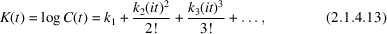where the k's are called the cumulants and may be regarded as being defined by the equation. They can be evaluated in terms of the moments by combining the series (2.1.4.10)for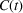with the ordinary series for the logarithm and equating the coefficients of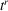. In most cases the process as described is tedious, but it can be shortened by use of a general method [Stuart & Ord (1994), Section 3.14, pp. 87–88; Exercise 3.19, p. 119]. Obviously, the cumulants exist only if the moments exist. The first few relations are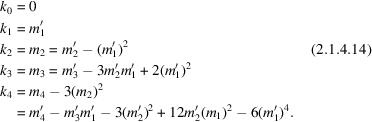Such expressions and their converses up to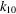are given by Stuart & Ord (1994, pp. 88–91). Since all the cumulants exceptcan be expressed in terms of the central moments only (i.e., those unprimed), onlyis changed by a change of the origin. Because of this property, they are sometimes called the semi-invariants (or seminvariants) of the distribution. Since addition of random variables is equivalent to the multiplication of their characteristic functions [equation (2.1.4.8)] and multiplication of functions is equivalent to the addition of their logarithms, each cumulant of the distribution of the sum of a number of random variables is equal to the sum of the cumulants of the distribution functions of the individual variables – hence the name cumulants. Although the cumulants (except) are independent of a change of origin, they are not independent of a change of scale. As for the moments, a change of scale simply multiplies them by a power of the scale factor; if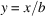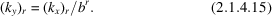The cumulants of the normal distribution are particularly simple. From equation (2.1.4.5), the cumulant-generating function of a normal distribution is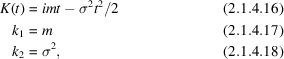all cumulants with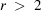are identically zero.

### ReferencesStuart, A. & Ord, K. (1994). Kendall's advanced theory of statistics. Vol. 1. Distribution theory, 6th ed. London: Edward Arnold.Google Scholar